Latest Banking jobs   »

# Quantitative Aptitude Quiz For RBI Grade B Phase 1 2023 -24th May

Q1. Sam is 20% less efficient than Anu & both complete the work together in 20 days. If another person Mohit takes 10 days more than Anu and Sam together to complete the same work. Then find in how many days all three complete the work together?
(a) 10 days
(b) 18 days
(c) None of these
(d) 12 days
(e) 15 days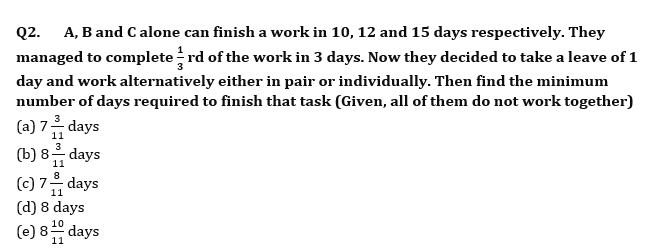Q3. A contractor gives a contract to ‘X’ for building a wall in 8 days. After 2 days of starting of work he realizes that he will be able to finish 64% of work in the contracted time, so he invite Y and Z due to which they complete the work on 6th day from starting. At the end, if ratio of money received by X, Y and Z is 12 : 8 : 5 then find in how many days Z can alone complete the work?
(a) 15 days
(b) 16 days
(c) 29 days
(d) 20 days
(e) None of these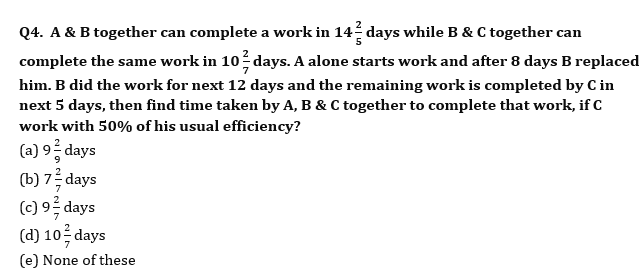Q5. A contractor was given a contract of completing a task in 60 days. He hired 40 men for completing the task. At the end of 36 days, he realized that only 50% task has been completed. So, in order to complete the task in the contracted time he hired more men. How many more men are hired by contractor to complete the task in contracted time?
(a) 20 men
(b) 18 men
(c) 25 men
(d) 12 men
(e) 16 men

Q6. A & B can complete a piece of work in 20 days. They both start working together and after 8 days, A got ill. Due to A’s sickness, A’s efficiency falls down by 40% and thus the total work completed in 24 days, then find in how many days B alone can complete the remaining work after A got sick.
(a) 31 days
(b) 34 days
(c) 20 days
(d) 32 days
(e) None of the above.

Q7. Anurag & Ayush together can do a work in 40/3 hours, while Veer alone can do the same work in ______ hours. Anurag & Veer start same work together and after four hours Anurag left the work, while Veer alone worked for three more hours, after that Ayush joined Veer and both complete the remaining work in _____hours. Ayush & Veer can complete the same work together in 22.5/2 hours, while efficiency of Anurag is 25% more than Ayush. Find the value of the blanks.
(a) 20 & 5
(b) 18 & 6
(c) 18 & 5
(d) 18 & 8
(e) 20 & 6

Q8. Arun daily works for X hours and earns Rs Y per hour. For each hour he works in excess of X hours, he is paid two times of his original rate. One day he works for Z hours and the amount he is paid on that day is Rs 1200 more than his daily amount. Find the possible value of (Z,X) if Y is either 100 or 150?
(i)12,8
(ii)10,4
(iii)9,5
(iv)13,7
(v)17,13
(a) Only (i) and (iii)
(b) Only (ii)and (iv)
(c) Only (ii) and (v)
(d) Only (i),(iii) and (v)
(e) All of the above

Q9. Simple interest for a period of two year is given as Rs. 1800 and the difference between CI and SI for two years is Rs. 270. Find the CI for three years given that sum is same and rate of interest is also same?
(a) None of these
(b) Rs. 3551
(c) Rs. 3135
(d) Rs. 2721
(e) Rs. 3591

Q10. Shivam invested Rs 3 lac in a scheme which is providing interest rate of ‘r’% per annum at CI and this scheme doubles the sum invested in 72/r years which is two times of rate of interest provided by the scheme. Find the total amount received by Shivam at the end of 48 years (in lakhs)?
(a) 45
(b) 50
(c) 64
(d) 32
(e) 48

Solutions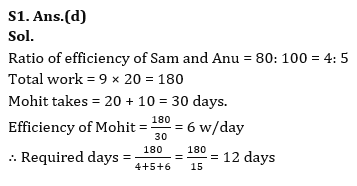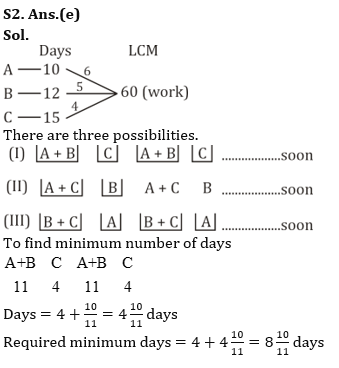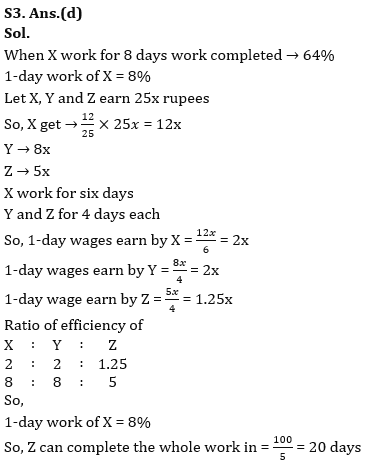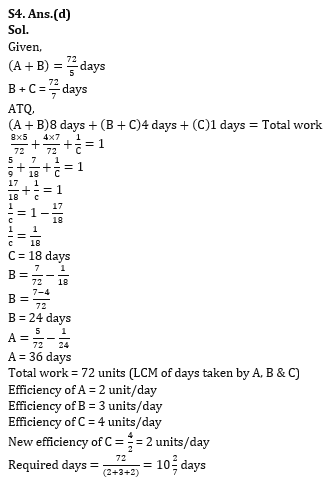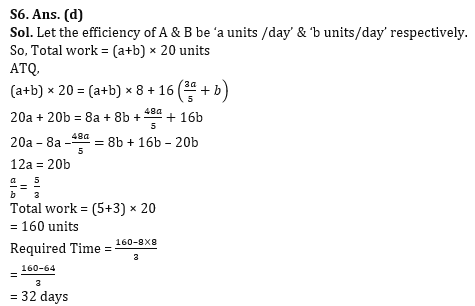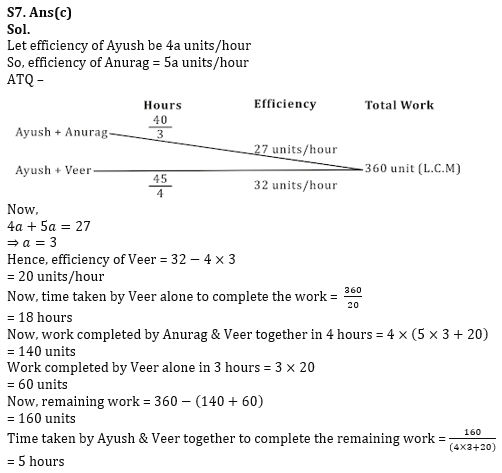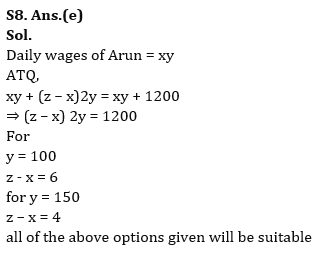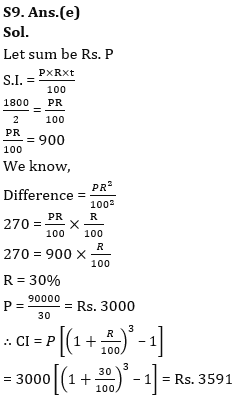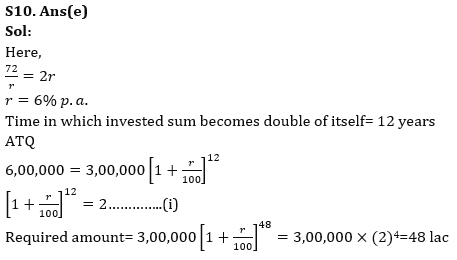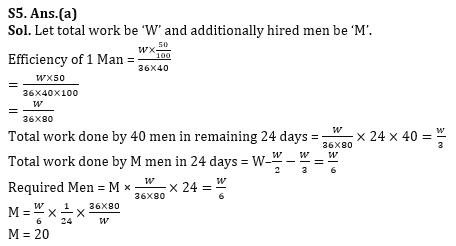## FAQs

### How many sections are there in the RBI Grade B Phase 1 Exam?

There are 4 sections in the RBI Grade B Phase 1 Exam i.e. English Language, General Awareness, Quantitative Aptitude & Reasoning.

#### Congratulations!Union Budget 2023-24: Free PDF